# SSAT Upper Level Math : How to graph a quadratic function

## Example Questions

### Example Question #5 : How To Graph A Quadratic Function

What is the vertex of the function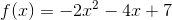?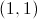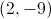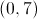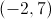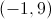Explanation: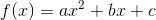The-coordinate of the vertex is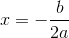, where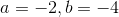.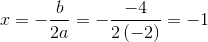To get the-coordinate, evaluate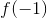.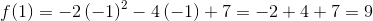The vertex is.